# Mensuration 2D

Mensuration 2D mainly deals with problems on perimeter and area. The shape is two dimensional, such as triangle, square, rectangle, circle, parallelogram, etc. This topic does not has many variations and most of the questions are based on certain fixed formulas.

• Perimeter : The length of the boundary of a 2D figure is called the perimeter.
• Area : The region enclosed by the 2D figure is called the area.
• Pythagoras Theorem : In a right angled triangle, (Hypotenuse)2 = (Base)2 + (Height)2

### Triangle

Let the three sides of the triangle be a, b and c.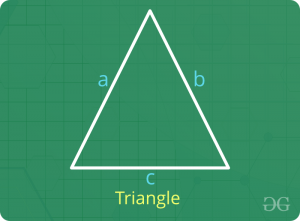• Perimeter = a + b + c
• Area
1. 2s = a + b + c
Area =2. Area = 0.5 x Base x Perpendicular Height

### Rectangle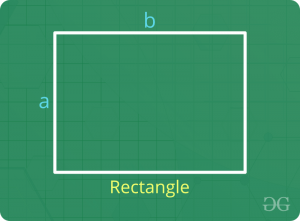• Perimeter = 2 x (length + Breadth)
• Area = Length x Breadth

### Square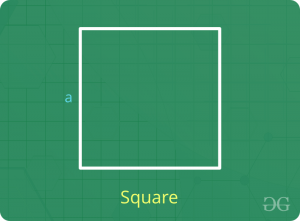• Perimeter = 4 x Side Length
• Area = (Side Length)2 = 0.5 x (Diagonal Length)2

### Parallelogram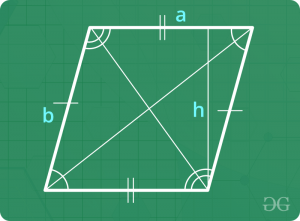• Perimeter = 2 x Sum of adjacent sides
• Area = Base x Perpendicular Height

### Rhombus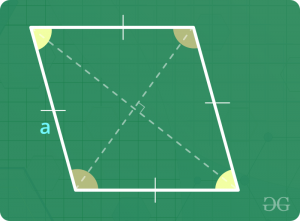• Perimeter = 4 x Side Length
• Area = 0.5 x Product of diagonals

### Trapezium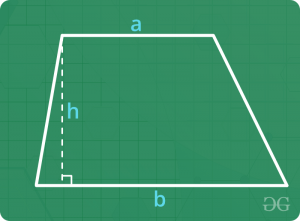• Perimeter = Sum of all sides
• Area = 0.5 x Sum of parallel sides x Perpedicular Height

### Circle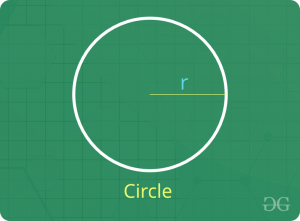• Perimeter = 2 π Radius
• Length of an arc that subtends an angle θ at the centre of the circle = (π x Radius x θ) / 180
• Area of a sector that subtends an angle θ at the centere of the circle = (π x Radius2 x θ) / 360

### Sample Problems

Question 1 : Find the perimeter and area of an isosceles triangle whose equal sides are 5 cm and height is 4 cm.
Solution : Applying Pythagoras theorem,
(Hypotenuse)2 = (Base)2 + (Height)2
=> (5)2 = (0.5 x Base of isosceles triangle)2 + (4)2
=> 0.5 x Base of isosceles triangle = 3
=> Base of isosceles triangle = 6 cm
Therefore, perimeter = sum of all sides = 5 + 5 + 6 = 16 cm
Area of triangle = 0.5 x Base x Height = 0.5 x 6 x 4 = 12 cm2

Question 2 : A rectangular piece of dimension 22 cm x 7 cm is used to make a circle of largest possible radius. Find the area of the circle such formed.
Solution : In questions like this, diameter of the circle is lesser of length and breadth.
Here, breadth Diameter of the circle = 7 cm
=> Radius of the circle = 3.5 cm
Therefore, area of the circle = π (Radius)2 = π (3.5)2 = 38.50 cm2

Question 3 : A pizza is to be divided in 8 identical pieces. What would be the angle subtended by each piece at the centre of the circle ?
Solution : By identical pieces, we mean that area of each piece is same.
=> Area of each piece = (π x Radius2 x θ) / 360 = (1/8) x Area of circular pizza
=> (π x Radius2 x θ) / 360 = (1/8) x (π x Radius2)
=> θ / 360 = 1 / 8
=> θ = 360 / 8 = 45
Therefore, angle subtended by each piece at the centre of the circle = 45 degrees

Question 4 : Four cows are tied to each corner of a square field of side 7 cm. The cows are tied with a rope such that each cow grazes maximum possible field and all the cows graze equal areas. Find the area of the ungrazed field.
Solution : For maximum and equal grazing, the length of each rope has to be 3.5 cm.
=> Area grazed by 1 cow = (π x Radius2 x θ) / 360
=> Area grazed by 1 cow = (π x 3.52 x 90) / 360 = (π x 3.52) / 4
=> Area grazed by 4 cows = 4 x [(π x 3.52) / 4] = π x 3.52
=> Area grazed by 4 cows = 38.5 cm2
Now, area of square field = Side2 = 72 = 49 cm2
=> Area ungrazed = Area of field – Area grazed by 4 cows
=> Area ungrazed = 49 – 38.5 = 10.5 cm2

Question 5 : Find the area of largest square that can be inscribed in a circle of radius ‘r’.
Solution : The largest square that can be inscribed in the circle will have the diameter of the circle as the diagonal of the square.
=> Diagonal of the square = 2 r
=> Side of the square = 2 r / 21/2
=> Side of the square = 21/2 r
Therefore, area of the square = Side2 = [21/2 r]2 = 2 r2

Question 6 : A contractor undertakes a job of fencing a rectangular field of length 100 m and breadth 50 m. The cost of fencing is Rs. 2 per metre and the labour charges are Re. 1 per metre, both paid directly to the contractor. Find the total cost of fencing if 10 % of the amount paid to the contractor is paid as tax to the land authority.
Solution : Total cost of fencing per metre = Rs. 2 + 1 = Rs. 3
Length of fencing required = Perimeter of the rectangular field = 2 (Length + Breadth)
=> Length of fencing required = 2 x (100 + 50) = 300 metre
=> Amount paid to the contractor = Rs. 3 x 300 = 900
=> Amount paid to the land authority = 10 % of Rs. 900 = Rs. 90
therefore, total cost of fencing = Rs. 900 + 90 = Rs. 990

### Problems on Mensuration 2D | Set 2

Programs on Triangle

Programs on Rectangle

Programs on Square

Programs on Parallelogram

Programs on Rhombus and Trapezium

Programs on Circle

Please write comments if you have any doubts related to the topic discussed above, or if you are facing difficulty in any question or if you would like to discuss a question other than those mentioned above.